Blog Post

# Using the Right Mean for Meaningful Performance Analysis

Learn how to choose the right mean to provide the most extensive and accurate insight into your performance data analysis here.

Performance analytics is a field which deals with huge discrete data sets that need to be grouped, organized, and aggregated to gain an understanding of the data. Synthetic and real user monitoring are the two most popular techniques to evaluate the performance of websites; both these techniques use historical data sets to evaluate performance.

In web performance analytics, it is preferred to use statistical values that describe a central tendency ( the odd numbermeasure of central location) for the discrete data set under observation. The statistical metric can be used to evaluate and analyze the data. These data sets have innumerable data points that need to be aggregated using different statistical approaches.

With the number of statistical metrics available, the big question is how do you determine the right statistical metric for a given data set. Mean, Median, and Geometric Mean are all valid measures of central tendency, but under different conditions, some measures of central tendency are more appropriate to use than others.

This article discusses different statistical approaches used in the world of web performance evaluation and the methods preferred in different contexts of performance analysis using real-world performance data.

### Common Statistical Metrics

• Arithmetic Mean (Average)

The average is used to describe a single central value in a large set of discrete data. The mathematical formula to calculate the average is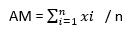The average is equal to the sum of all data points divided by the number of items, where ‘n’ represents the number of data samples.

• Median

Median is the middle score for a set of data that has been arranged in the order of magnitude. Let us consider a set of data point as [12, 31, 44, 47, 22, 18, 60, 75, 80]. To get the median of the data set the data points need to be sorted in ascending order.

12, 18, 22, 31, 44, 47, 60, 75, 80

The median for the above data set is ’44’ as the middle item is (n+1)/2 if odd number of items. The median would be n/2 if there is even number of items in the series.

• Geometric Mean

Geometric mean is the nth positive root of the product of n positive given values. The mathematical formula to calculate the geometric mean for X containing n discrete set of data points is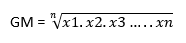• Standard Deviation

Standard deviation is used for measuring the extent of variation of the data samples around the center. The mathematical formulae to calculate the standard deviation for a set of data samples is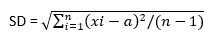Where ‘a’ denotes the average of ‘n’ data samples of value ‘x’.

### Determining the Right Statistical Approach

The two graphs below illustrate the different data distributions we come across in web performance monitoring. Using the formulae explained above, we have derived the average, median and the geometric mean of the webpage load time for website A and B.

##### Webpage load time Website A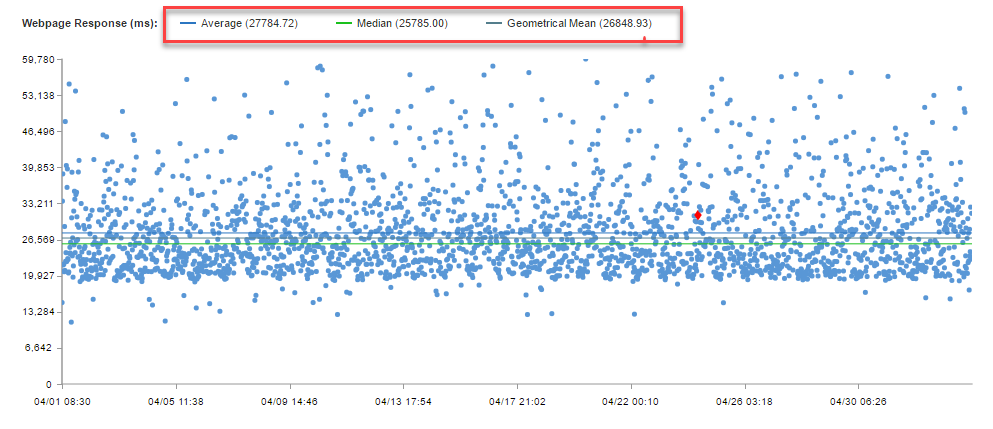##### Webpage load time Website B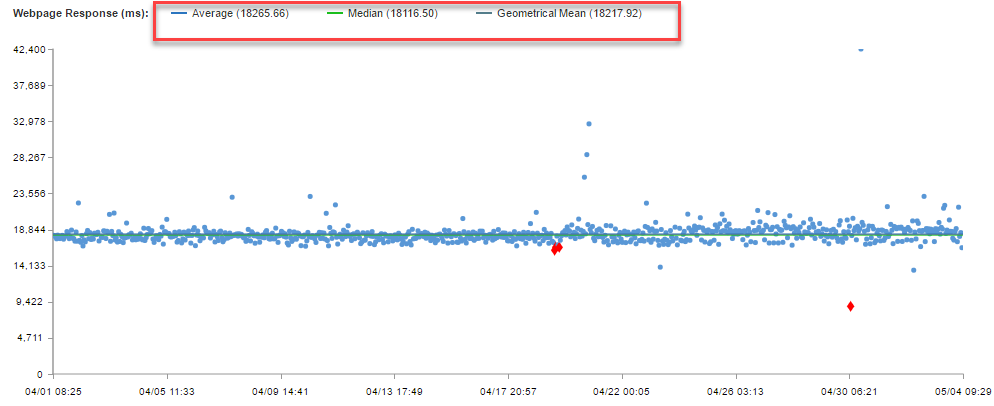Let us discuss a few use cases to understand how different statistical metrics are applicable in different scenarios.

### USE CASE 1

##### G1 – Scatter plot showing webpage load time data set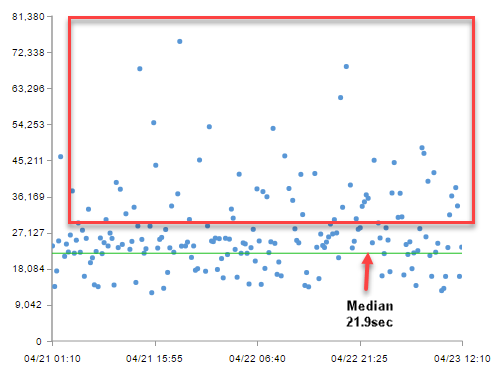##### G2 – Histogram shown the distribution of data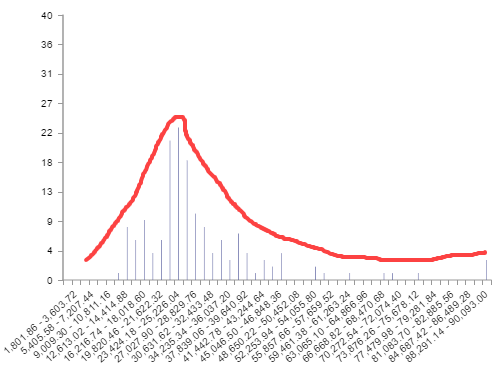The graphs G1 and G2 plots data for webpage load time. The uneven distribution of the data points in the scatterplot and histogram helps us understand how inconsistent the load time is.

We can see a higher number of data points in the trailing end of the Gaussian distribution in the histogram (G2); this means that most of the data points are of higher value.

What would be a good statistical metric in such cases? Before answering this, lets us take an example. Consider the following data set

Data Set = [4,4.3,5,6.5,6.8,7,7.2,20,30]

If we use median it gives a value of 6.8. But most of the data points tend towards a higher range with 30 being the highest. So, taking the median value in cases with higher outliers is not an accurate estimate of the page load time. Median should be used for data sets with fewer outliers and values that are concentrated towards the center of the Gaussian distribution.

Now let us take the average for this same data set. This gives us a value of 27.4 which is slightly more skewed towards the outlier values. Once again, the average is not an accurate measure for web page load time.

Since median and average don’t apply to this set of data, let us consider the geometric mean. We get a value of 7.8 using geometric mean; this value is closer to the central value and is not skewed to the higher or lower values in the data set.

In this use case, we have determined the geometric mean as the most accurate statistical method to analyze the data.

### USE CASE 2

##### G3 – Scatter plot showing webpage load time data set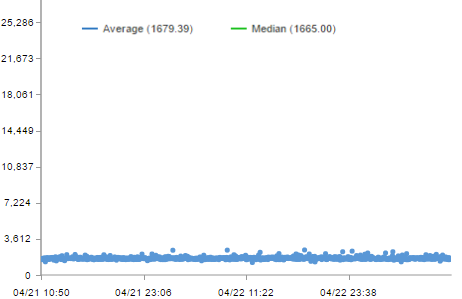##### G4 – Histogram shown the distribution of data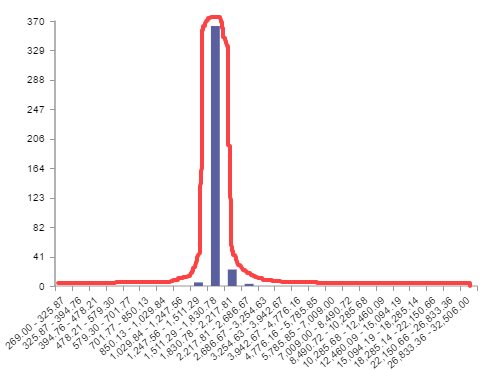In the graphs above (G3 and G4), most of the data points are close to each other with a higher population in the center of Gaussian surface. The difference between each of the data points are much less than the distribution considered in the previous scenario. This indicates a consistent page load time across different test runs.

Using average or median to evaluate the central tendency would be more accurate in this case as there are not many outliers so the average wouldn’t be skewed towards the outlier values.

### USE CASE 3

##### Website A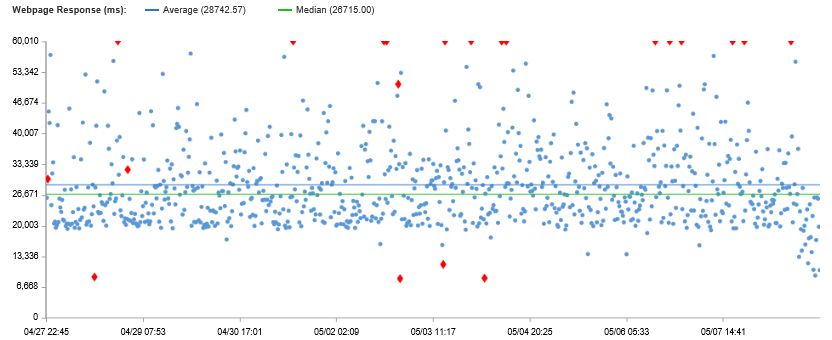##### Website B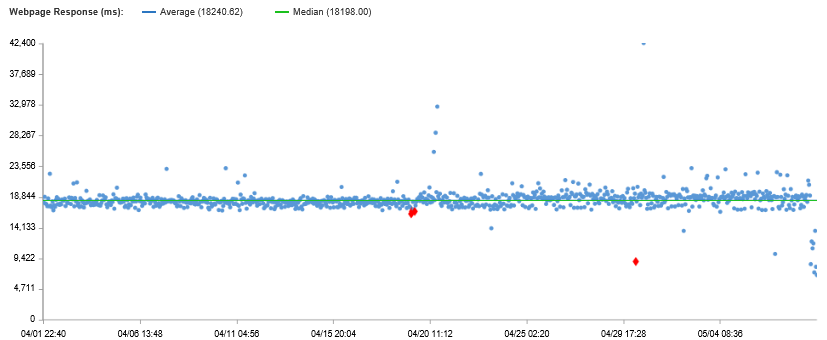The above data distribution shows the webpage load time for two different websites. In performance analysis, we need to evaluate the consistency of a webpage. And if there is high volatility in the page performance then we should be able to measure the difference between the central value versus the outliers.

In this case, the standard deviation values are 9.1 and 1.7 seconds for website A and B respectively while the median for website A and B are 26.6 and 18.1 seconds. Based on the standard deviation values, we see there are data points for website A at 36 secs (median + SD) and website B at 20 secs (median + SD). This means that website A had high number of data points concentrated at 36 secs or more and website B had high number data points concentrated at 20 secs or more.

To know what percent of data had higher value when compared to the standard deviation we can use the cumulative distribution graph.

##### Website A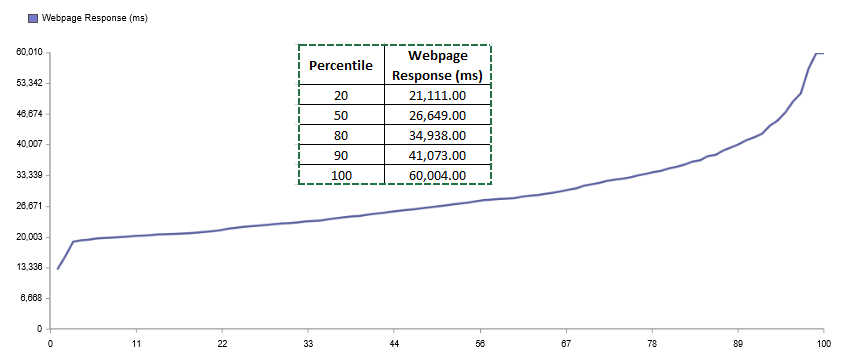##### Website B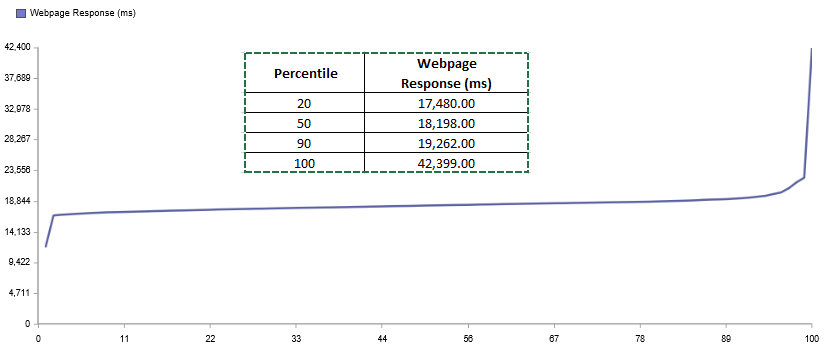From the cumulative distribution graph shown above we can see that website A had almost 20% of data points higher than the standard deviation values whereas website B had 10% of data more than standard deviation value.

Standard deviation can be used for evaluating how far and consistent the data points are with respect to the central value of data distribution in performance analysis.

Median and average are applicable when the data points are concentrated towards the center of the Gaussian distribution. On the other hand, if there are more data points distributed towards the tail of the Gaussian distribution and there is a high difference between each data point then geometric mean would be a better choice. Standard deviation should be used to understand the variance of the data points from the median value and to gauge the consistency of the sites performance.

Synthetic Monitoring
Real User Monitoring
SLA Management
Media and Entertainment

Blog post

Blog post

Blog post